Prev: 6.1.4 Rate Calculation Up: 6.1 Modeling of Low-Pressure Next: 6.2 Low-Pressure Processes

## 6.1.5 Surface Propagation

The final step for the profile evolution is the surface propagation perpendicular to the surface according to the previously calculated surface velocity. One possibility for the calculation of the normal component of the rate is the usage of distribution functions already expressed with respect to the surface normal. This can be achieved by multiplying the distribution functions with the cosine of the incidence direction, which is the usual procedure for segment-based algorithms.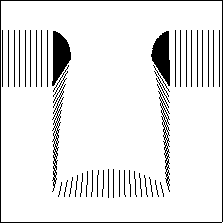Within our morphologically based approach a vector integration of the distribution functions is applied which leads to a rate vector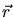whose components are given as floating point value. This value is mapped to structuring elements. Their main axis is given by the direction ofand the length of the main axis equals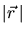, resolved within the cellular geometry representation. The minor axis is given by the isotropic component of the rate. The application of aligned structuring elements is geometrically equivalent to the multiplication with the cosine of the incidence direction.

Fig. 6.11 shows the rate vectors calculated for sputter deposition into a cylindrical via at a center wafer position. The rate vectors are obtained by vector integration of a cosine function assumed as distribution of the incident particles.

As explained in Section 2.2 the outer contour of the structuring elements applied at all surface positions forms the profile for a new time-step in the surface propagation procedure. In the general case ellipsoidals are used as structuring elements. Their main axes are given by the directional rate, the minor axes are given by the isotropic rate and are only of secondary importance. The envelope of all ellipsoidals representing the evolving surface is determined by the main axes of the elements. For the purpose of algorithmic optimization and acceleration the minor axes can be diminished to the thickness of one cell width used for the volume discretization, degenerating the ellipsoidals to lines. This method is similar to the one described in Section 2.3.2 for the optimization of isotropic processes by means of linear structuring elements and leads to a comparable acceleration in the surface propagation algorithm.

Since the rate integration is carried out for each surface cell, the density of the linear structuring elements given by the rate vectors (see Fig. 6.11) is high enough to guarantee that the new surface is smooth and continuous. Special care has to be taken at sharp corners of the geometry, where an interpolation between the rate vectors of two adjacent surface cells is necessary (cf. Fig. 6.11).

Finally, we have taken all steps in order to run simulations: By means of solid modeling we can generate any desired input geometry, we have introduced the algorithm for the surface propagation, the required equations for the rate calculations are derived, and reactor scale effects are considered.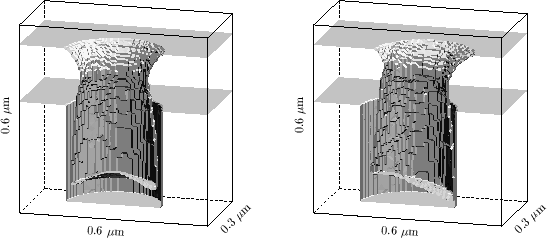Figure 6.12 shows the simulation results of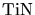sputter deposition into a 0.3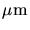diameter, 0.3deep circular hole located 260mm below the sputter target disk according to the geometry from Fig. 6.4. For both simulations the exponential distribution function from (6.4) is used, the offset for the angle of maximum incidence is 12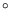, resulting in a distribution similar to the one on the right hand side of Fig. 6.5. The deposition rate is 33.8nm/min and the deposition time is 320s.

The figure on the left hand side shows the resulting profile for a contact hole in the center of the wafer. As expected the resulting film profile is radially symmetric. The characteristics of the profile, namely, the overhang at the top edge of the via, the low sidewall coverage, and the increased thickness in the center of the bottom of the via are typical for this kind of sputter process. If higher aspect ratio vias are to be filled, the directionality of this process might be insufficient and different techniques like collimated sputtering or ionized magnetron sputtering  have to be applied.

On the right hand side, the deposition at a position 90mm off the wafer center was performed by a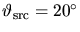azimuthal offset of the distribution function.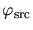was set to 90so that the center of the sputter target is situated on the right hand side of the structure. The asymmetric profile reflects the slanted angle of principle particle incidence. The left sidewall is oriented towards the target whereas for the right sidewall most of the incident particles are shadowed. This causes strong variations in the sidewall coverage, which depend on the orientation towards the direction of main particle incidence.

With this illustrative example the basics of the low-pressure processes have been summarized. In the next section, the considerations will be extended and adapted in order to model typical low-pressure etching and deposition techniques applied in semiconductor manufacturing.

W. Pyka: Feature Scale Modeling for Etching and Deposition Processes in Semiconductor Manufacturing